# Big Ideas Math Geometry Chapter 6: Relationships Within Triangles Chapter Exam

Exam Instructions:

Choose your answers to the questions and click 'Next' to see the next set of questions. You can skip questions if you would like and come back to them later with the yellow "Go To First Skipped Question" button. When you have completed the practice exam, a green submit button will appear. Click it to see your results. Good luck!

### Page 2

#### Question 7 7. In the pictured triangle, XV is an angle bisector. What is the length of XY?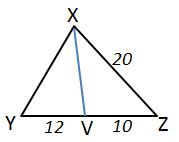#### Question 8 8. In the pictured triangle, FE is an angle bisector. What is the length of GE?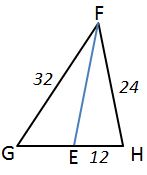#### Question 9 9. In the pictured triangle, MS is an angle bisector. What is the length of SO?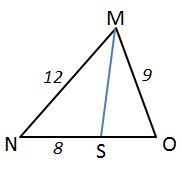#### Question 10 10. A segment AB is divided by a perpendicular bisector, as shown in the picture. The perpendicular bisector crosses the segment at point C. If it is 3.5 inches from point A to point C, how long is the entire segment?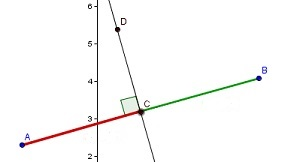### Page 3

#### Question 14 14. In the pictured triangle, AD is an angle bisector. What is the length of AC?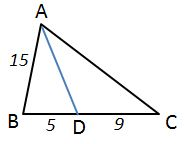### Page 4

#### Question 16 16. Which of the following represents the angle bisector theorem for the pictured triangle?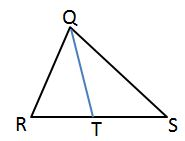#### Question 17 17. A segment AB is divided by a perpendicular bisector, as shown in the picture. The perpendicular bisector crosses the segment at point C. If it is 18 inches from point A to point B, how long is the distance from point A to point C?### Page 5

#### Big Ideas Math Geometry Chapter 6: Relationships Within Triangles Chapter Exam Instructions

Choose your answers to the questions and click 'Next' to see the next set of questions. You can skip questions if you would like and come back to them later with the yellow "Go To First Skipped Question" button. When you have completed the practice exam, a green submit button will appear. Click it to see your results. Good luck!

Support